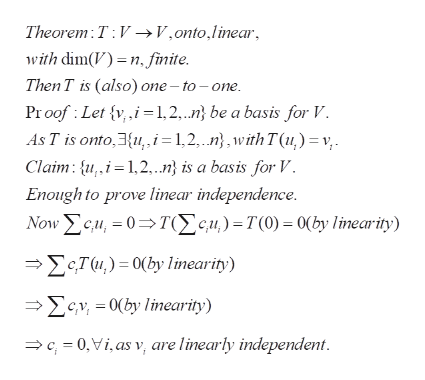# There exists a linear transformation T:IR^2019->IR^2019 that is onto but not one-to-one. Is this true or false?

Question
2 views

There exists a linear transformation T:IR^2019->IR^2019 that is onto but not one-to-one. Is this true or false?

check_circle

Step 1

To determine whether the given statement about linear transformations is true or not

Step 2

The number 2019 is immaterial. We will prove that the statement is FALSE. (not only fo r2019, but for any positive integer n).

Step 3

Let n be a positive integer and V be a finite dimensional vector space. Then T is a linear transfor...help_outlineImage TranscriptioncloseTheorem T V>V,onto,linear, with dim()n, finite. Then T is (also) one -to-one Proof Let vi=1,2,..n} be a basis for V. As T is onto,3{u, i= 1,2,..n},with T(u) v Claim: i-1,2.n} is a basis for V. Enough to prove linear independence. Now cu,0Tcu) =T(0) = 0(by linearity) - ΣςΤα) = 0(ν linearip) e0(by linearity) c0,Vi, as v, are linearly independent. fullscreen

### Want to see the full answer?

See Solution

#### Want to see this answer and more?

Solutions are written by subject experts who are available 24/7. Questions are typically answered within 1 hour.*

See Solution
*Response times may vary by subject and question.
Tagged in

### Math